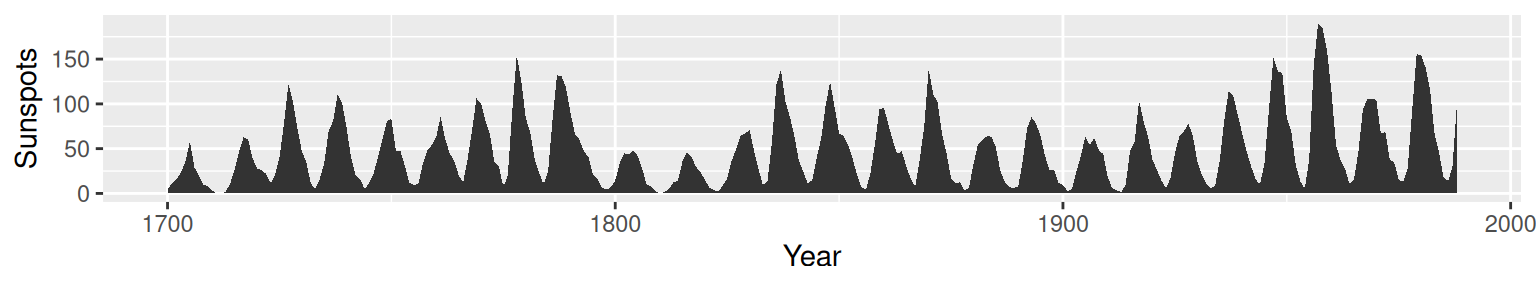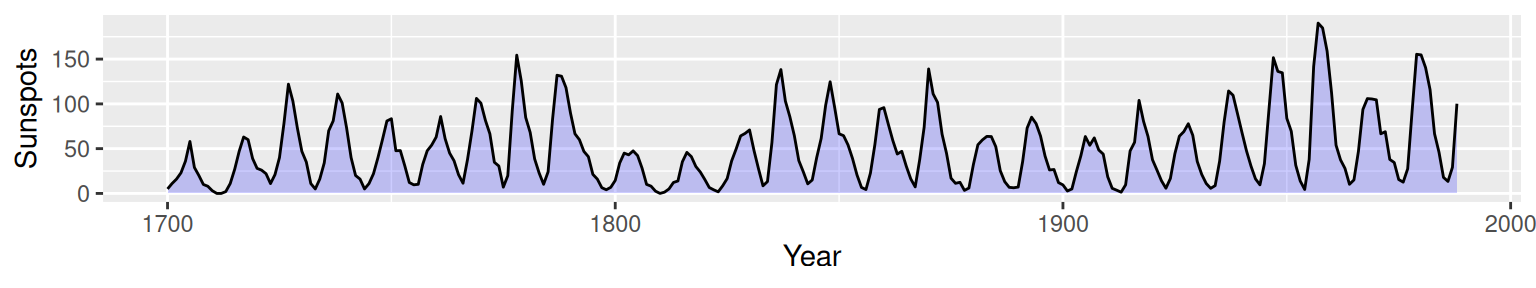## 4.6 Making a Graph with a Shaded Area

### 4.6.1 Problem

You want to make a graph with a shaded area.

### 4.6.2 Solution

Use `geom_area()` to get a shaded area, as in Figure 4.17:

``````# Convert the sunspot.year data set into a data frame for this example
sunspotyear <- data.frame(
Year     = as.numeric(time(sunspot.year)),
Sunspots = as.numeric(sunspot.year)
)

ggplot(sunspotyear, aes(x = Year, y = Sunspots)) +
geom_area()``````Figure 4.17: Graph with a shaded area

### 4.6.3 Discussion

By default, the area will be filled with a very dark grey and will have no outline. The color can be changed by setting `fill`. In the following example, we’ll set it to `"blue"`, and we’ll also make it 80% transparent by setting `alpha` to 0.2. This makes it possible to see the grid lines through the area, as shown in Figure 4.18. We’ll also add an outline, by setting colour:

``````ggplot(sunspotyear, aes(x = Year, y = Sunspots)) +
geom_area(colour = "black", fill = "blue", alpha = .2)``````Figure 4.18: Graph with a semitransparent shaded area and an outline

Having an outline around the entire area might not be desirable, because it puts a vertical line at the beginning and end of the shaded area, as well as one along the bottom. To avoid this issue, we can draw the area without an outline (by not specifying colour), and then layer a `geom_line()` on top, as shown in Figure 4.19:

``````ggplot(sunspotyear, aes(x = Year, y = Sunspots)) +
geom_area(fill = "blue", alpha = .2) +
geom_line()``````Figure 4.19: Line graph with a line just on top, using `geom_line()`